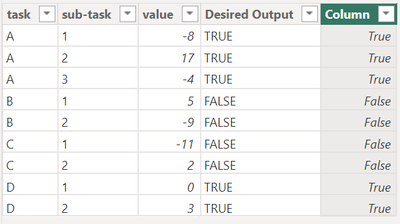cancel
Showing results for
Did you mean:Helper III

## Label as True/False all the rows belonging to a higher level attribute, based on the sum of values

I would like to create a column formula that will label all the rows for an attribute either as True or False, based on the sum of values/net value resulting from other sub-attributes. Example: task A has a net value of its subtasks as +5, so all the rows belonging to task A would be a TRUE. Task B has a negative value of its sub-tasks as -4, so it would be a FALSE; and so on. The idea is to be able to filter out Tasks with a negative net value:

 task sub-task value Desired Output A 1 -8 TRUE A 2 17 TRUE A 3 -4 TRUE B 1 5 FALSE B 2 -9 FALSE C 1 -11 FALSE C 2 2 FALSE D 1 0 TRUE D 2 3 TRUE

Thank you.

2 ACCEPTED SOLUTIONSSuper User

Hey @tomekm ,

the below DAX can be used to create a calculated column:

``````Column =
SUMX(
)
return
IF( sumOfSubTasks > 0 , TRUE() , FALSE() )``````

A screenshot of the table:Hopefully, this provides what you are looking for.

Regards,

Tom

Did I answer your question? Mark my post as a solution, this will help others!

Proud to be a Super User!
I accept Kudos 😉
Hamburg, GermanyMemorable Member

Hi @tomekm ,
Try to add column with formula:

test = if(calculate(sum('Table (2)'[value]),ALLEXCEPT('Table (2)','Table (2)'[task]))>0,TRUE(),False).
I have attached a picture for your reference11 REPLIES 11Memorable Member

Hi @tomekm ,
Try to add column with formula:

test = if(calculate(sum('Table (2)'[value]),ALLEXCEPT('Table (2)','Table (2)'[task]))>0,TRUE(),False).
I have attached a picture for your referenceHelper III

Thanks. How can I modify this formula so that it works when a specific filter is applied in the report? For example, there is a "Status" column for the sub-tasks that labels them with values of: current, past, future. I want to be able to filter on sub-tasks with only 1 or 2 specific Statuses, and the TRUE or FALSE formula to perform the calculation based on those filter selections in the report.Super User

Hi,

Write these measures

``V = SUM(Data[value])``
``Measure = CALCULATE([V],ALL(Data[sub-task]))>0``

Hope this helps.Regards,
Ashish Mathur
http://www.ashishmathur.comHelper III

Thanks Ashish, but it's still not working in the context of filtering. For example, for task A, if sub-tasks 1 and 3 are labelled as 'previous' and sub-task 2 as 'current', and if I then filter on 'previous' only, I'd like to see those 2 entries labelled as FALSE, since they are net negative. And if I filter on 'current' subtasks only, I would want to see sub-task 2 for task A, and labelled as TRUE. Any ideas?Super User

You are welcome.  Share the download link of the PBI file, show the problem and the expected result very clearly.

Regards,
Ashish Mathur
http://www.ashishmathur.comHelper III

Thanks for the follow up, Ashish. I no longer need this, as the first solutions posted earlier work for me fine.Helper III

Thank you!Super User

Hey @tomekm ,

the below DAX can be used to create a calculated column:

``````Column =
SUMX(
)
return
IF( sumOfSubTasks > 0 , TRUE() , FALSE() )``````

A screenshot of the table:Hopefully, this provides what you are looking for.

Regards,

Tom

Did I answer your question? Mark my post as a solution, this will help others!

Proud to be a Super User!
I accept Kudos 😉
Hamburg, GermanyHelper III

Thanks. How can I modify this formula so that it works when a specific filter is applied in the report? For example, there is a "Status" column for the sub-tasks that labels them with values of: current, past, future. I want to be able to filter on sub-tasks with only 1 or 2 specific Statuses, and the TRUE or FALSE formula to perform the calculation based on those filter selections in the report.Super User

Hey @tomekm ,

instead of a calculated column you need a measure, as a measure adapts to user interactions.

But you have to be aware that it's not possible to use a measure inside a slicer.
I suggest that you create a measure that returns 1 for ge 0 and 0 for lt 0.

You can use the measure in combination with a visual level filter.

Hopefully, this provides an idea of how to tackle your challenge.

Regards,

Tom

Did I answer your question? Mark my post as a solution, this will help others!

Proud to be a Super User!
I accept Kudos 😉
Hamburg, GermanyHelper III

Thank you!